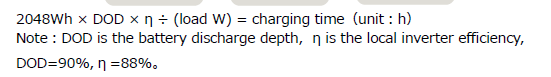# AC200Max AMPERAGE Remaining

Own a AC200Max. Is there a way to find out the number of AMPs that are remaining in the AC200Max?
Using the AC200Max to power a 12V diesel heater. Want to know the AMP hours remaining in the AC200Max so that remaining run time can be calculated.
Example. AC200Max has 166 AMPs. Therefore if 5 AMPs an hour is being consumed then 166 divided by 5 translates into the heater running for 33 hours.
Thx, kevin

No - but you can do the calculation with kWh.

So the AC200Max has a 2kWh battery. Assume 90% efficient = 1.8kWh or 1800 Wh.

5A * 12V = 60W so 1800 / 60 = 30 hours.

The Bluetti will give you % remaining, although it will not be 100% accurate, so you could say 50% remaining is 1kWh @ 90% efficiency = 900Wh.

@kevin , AC200Max has a capacity of 2048Wh, you can estimate the discharge time according to the following formula.Thanks for the response. It gives me the info that is needed.

Got it. Thanks. That answer’s my question.